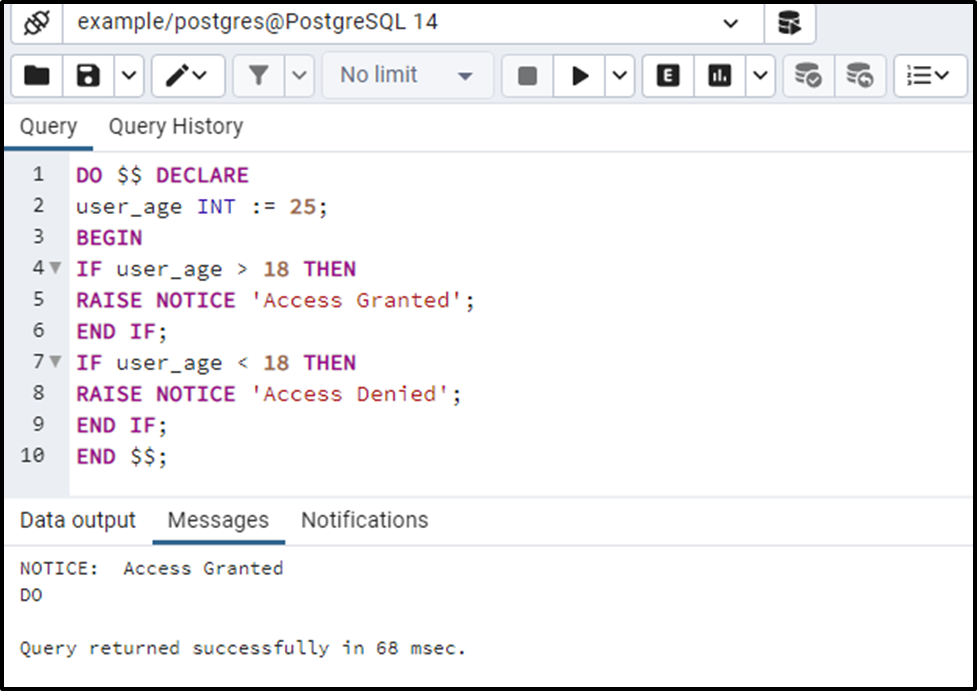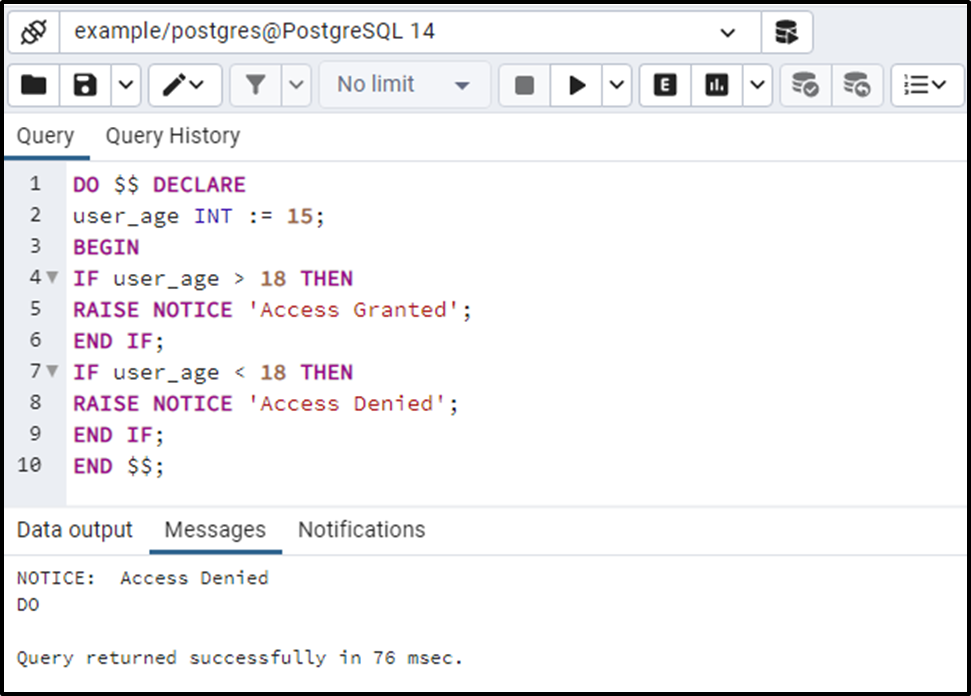##### PostgreSQL IF Statement With Examples

PostgreSQL provides some statements that assist users in decision-making scenarios. These statements take a decision on some specific criteria/condition and are hence referred to as control statements. In Postgres, the if-statement is one of the most valuable and frequently used control statements. The If statement executes only those expressions that satisfy the specified condition.

This post will present a thorough overview of the PostgreSQL if-statement with examples. So, let’s begin.

How to Use IF Statement in PostgreSQL?

In Postgres, the IF-statement only treats the true condition. The syntax of the IF statement is as follows:

```IF condition
THEN statement;
END IF;```

Here, condition represents a criterion that must be satisfied to execute the statements specified within the THEN block. If an expression doesn’t satisfy the specified criteria, then the if-statement moves the control to the next statement.

Example #1: How Does the IF Statement Work in PostgreSQL?

Let’s consider a scenario to understand the working of the IF statement:

```DO \$\$ DECLARE
user_age INT := 25;
BEGIN
IF user_age > 18 THEN
RAISE NOTICE 'Access Granted';
END IF;
IF user_age < 18 THEN
END IF;
END \$\$;```

- Firstly, we utilized the DO statement which indicates that we are going to execute all the statements that come under the DO statement.

- Next, we declared a variable user_age and assigned it a value 25.

- After we utilized the BEGIN statement to start the execution.

- Next, we utilized the IF statement that checks if the user_age > 18. If yes, then show a message “Access granted”.

- If no, then the control will be moved to the next statement after the END IF where the variable’s value will be tested against the second condition i.e. user_age < 18.The output authenticates the working of the if statement as it produced the accurate results.

Example #2: Working of IF statement in PostgreSQL?

Let’s modify the variable’s value and see how the if-statement work in that case:

```DO \$\$ DECLARE
user_age INT := 15;
BEGIN
IF user_age > 18 THEN
RAISE NOTICE 'Access Granted';
END IF;
IF user_age < 18 THEN
END IF;
END \$\$;```

This time the variable’s value will be tested against the first condition i.e. user_age > 18.

- The if-statement will return false, so the statements associated with the first condition will be skipped.

- The control will be moved to the second condition i.e. user_age<18.

- The if statement will compare the user’s age with the specified condition, i.e. 15 < 18.

- Consequently, it will return the following output: